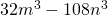Chapter 7: Factoring

# 7.5 Factoring Special Products

Now transition from multiplying special products to factoring special products. If you can recognize them, you can save a lot of time. The following is a list of these special products (note that a2 + b2 cannot be factored):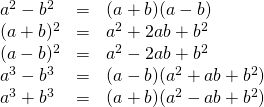The challenge is therefore in recognizing the special product.

Example 7.5.1

Factor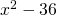.

This is a difference of squares.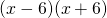is the solution.

Example 7.5.2

Factor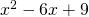.

This is a perfect square.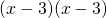or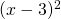is the solution.

Example 7.5.3

Factor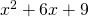.

This is a perfect square.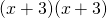or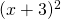is the solution.

Example 7.5.4

Factor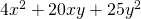.

This is a perfect square.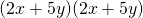or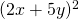is the solution.

Example 7.5.5

Factor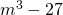.

This is a difference of cubes.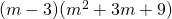is the solution.

Example 7.5.6

Factor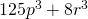.

This is a difference of cubes.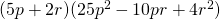is the solution.

# Questions

Factor each of the following polynomials.

1.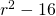2.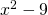3.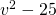4.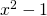5.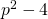6.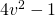7.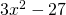8.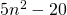9.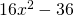10.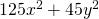11.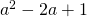12.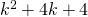13.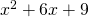14.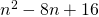15.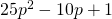16.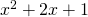17.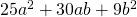18.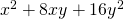19.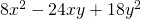20.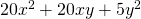21.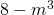22.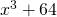23.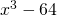24.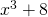25.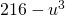26.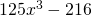27.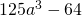28.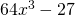29.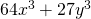30.